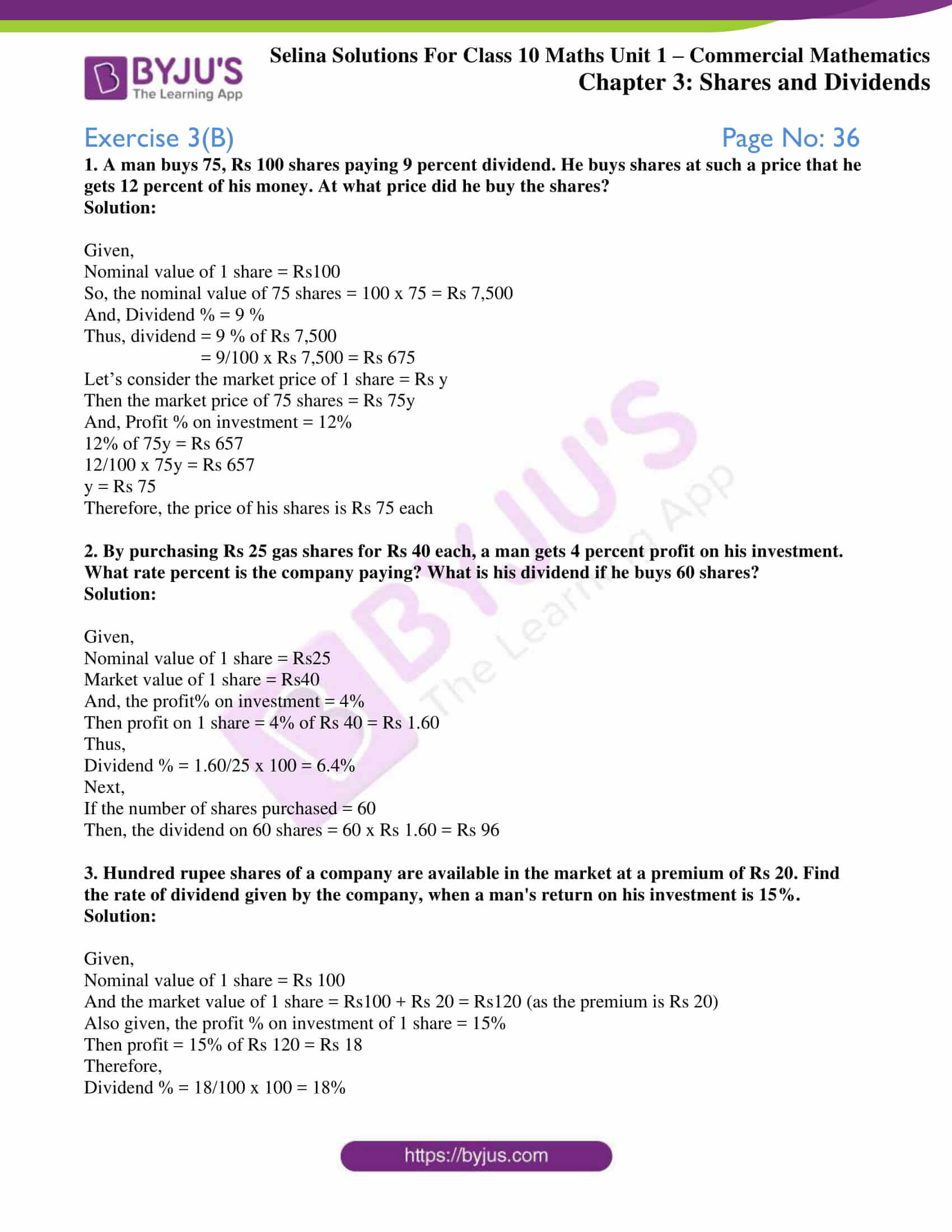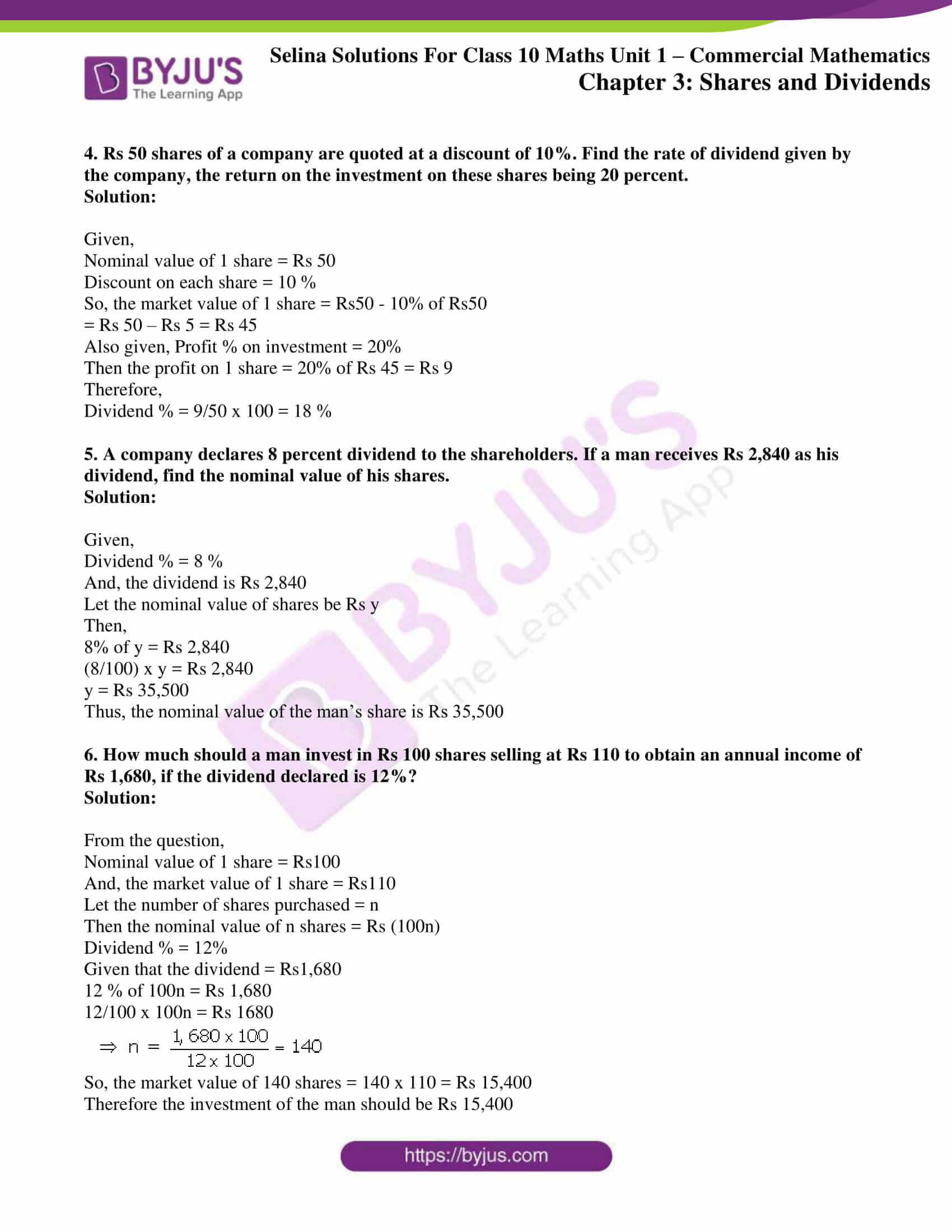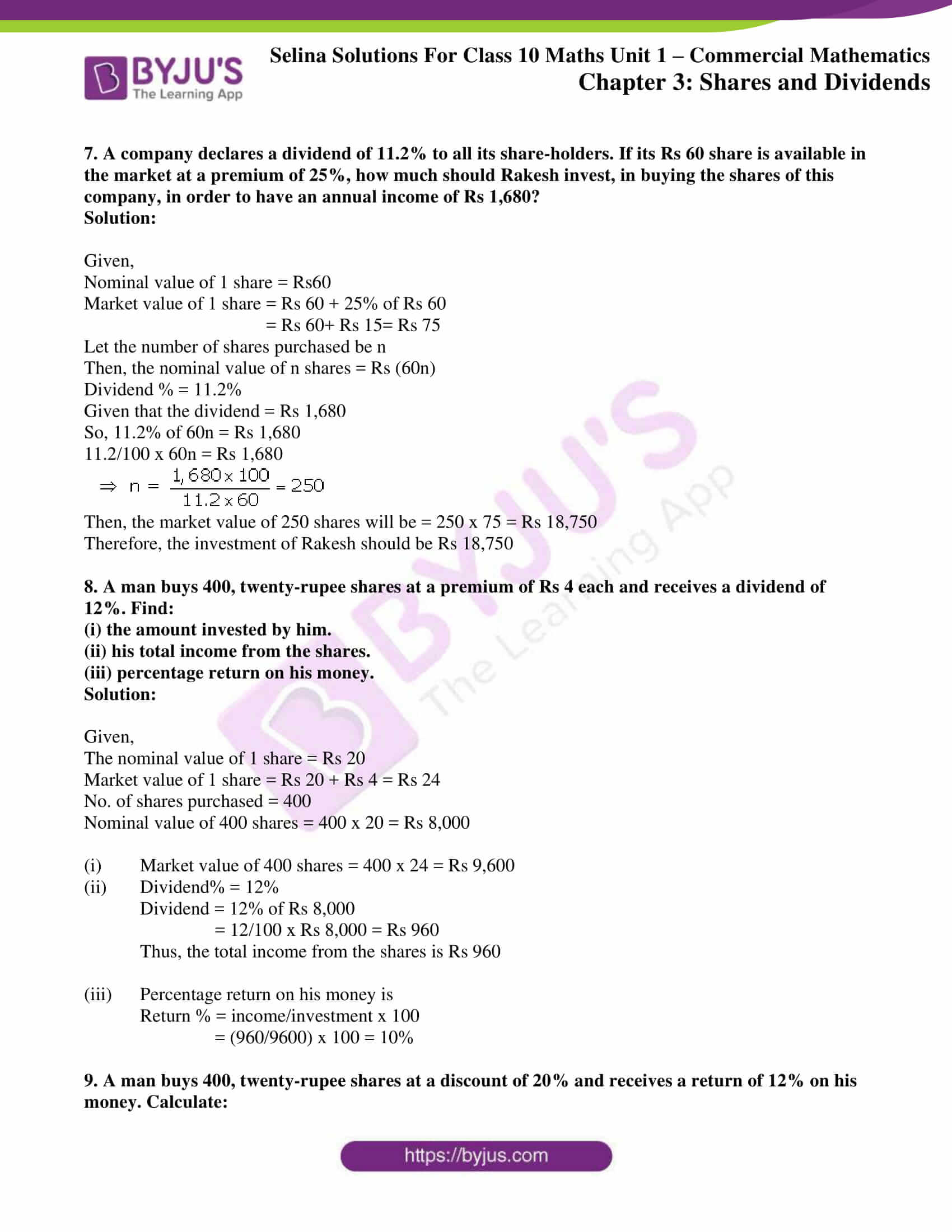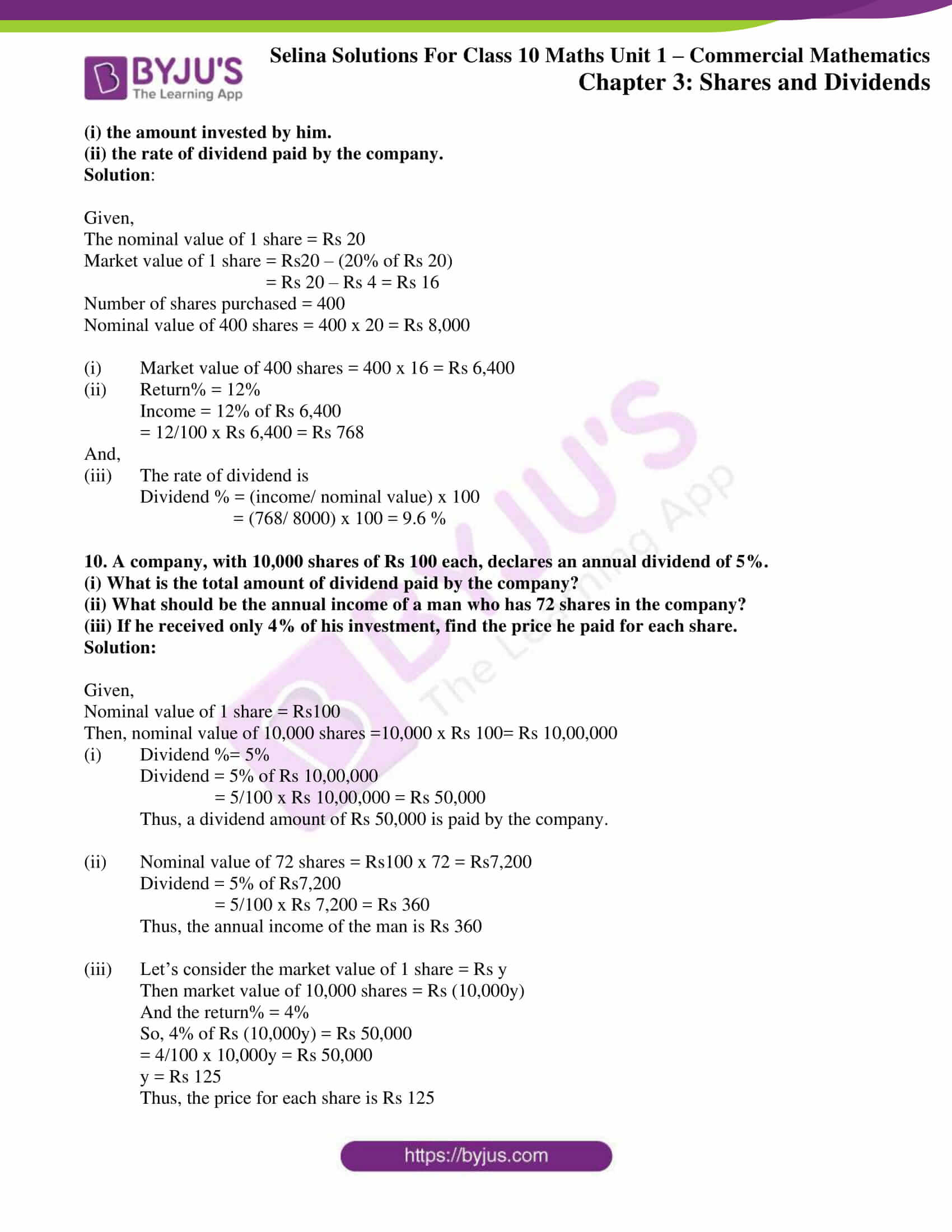# Selina Solutions Concise Maths Class 10 Chapter 3 Shares and Dividends Exercise 3(B)

Income from shares and dividends are a source of passive income. In this exercise, students will solve problems on calculating the rate of investment, declared dividend and comparing investments. For all the doubts a student has, the Selina Solutions for Class 10 Maths is the right place to get everything cleared. Students wanting to get the answers of this exercise can access the Concise Selina Solutions for Class 10 Maths Chapter 3 Shares and Dividends Exercise 3(B) PDF provided below.

## Selina Solutions Concise Maths Class 10 Chapter 3 Shares and Dividends Exercise 3(B) Download PDF### Access Selina Solutions Concise Maths Class 10 Chapter 3 Shares and Dividends Exercise 3(B)

1. A man buys 75, Rs 100 shares paying 9 percent dividend. He buys shares at such a price that he gets 12 percent of his money. At what price did he buy the shares?

Solution:

Given,

Nominal value of 1 share = Rs100

So, the nominal value of 75 shares = 100 x 75 = Rs 7,500

And, Dividend % = 9 %

Thus, dividend = 9 % of Rs 7,500

= 9/100 x Rs 7,500 = Rs 675

Let’s consider the market price of 1 share = Rs y

Then the market price of 75 shares = Rs 75y

And, Profit % on investment = 12%

12% of 75y = Rs 657

12/100 x 75y = Rs 657

y = Rs 75

Therefore, the price of his shares is Rs 75 each

2. By purchasing Rs 25 gas shares for Rs 40 each, a man gets 4 percent profit on his investment. What rate percent is the company paying? What is his dividend if he buys 60 shares?

Solution:

Given,

Nominal value of 1 share = Rs25

Market value of 1 share = Rs40

And, the profit% on investment = 4%

Then profit on 1 share = 4% of Rs 40 = Rs 1.60

Thus,

Dividend % = 1.60/25 x 100 = 6.4%

Next,

If the number of shares purchased = 60

Then, the dividend on 60 shares = 60 x Rs 1.60 = Rs 96

3. Hundred rupee shares of a company are available in the market at a premium of Rs 20. Find the rate of dividend given by the company, when a man’s return on his investment is 15%.

Solution:

Given,

Nominal value of 1 share = Rs 100

And the market value of 1 share = Rs100 + Rs 20 = Rs120 (as the premium is Rs 20)

Also given, the profit % on investment of 1 share = 15%

Then profit = 15% of Rs 120 = Rs 18

Therefore,

Dividend % = 18/100 x 100 = 18%

4. Rs 50 shares of a company are quoted at a discount of 10%. Find the rate of dividend given by the company, the return on the investment on these shares being 20 percent.

Solution:

Given,

Nominal value of 1 share = Rs 50

Discount on each share = 10 %

So, the market value of 1 share = Rs50 – 10% of Rs50

= Rs 50 – Rs 5 = Rs 45

Also given, Profit % on investment = 20%

Then the profit on 1 share = 20% of Rs 45 = Rs 9

Therefore,

Dividend % = 9/50 x 100 = 18 %

5. A company declares 8 percent dividend to the shareholders. If a man receives Rs 2,840 as his dividend, find the nominal value of his shares.

Solution:

Given,

Dividend % = 8 %

And, the dividend is Rs 2,840

Let the nominal value of shares be Rs y

Then,

8% of y = Rs 2,840

(8/100) x y = Rs 2,840

y = Rs 35,500

Thus, the nominal value of the man’s share is Rs 35,500

6. How much should a man invest in Rs 100 shares selling at Rs 110 to obtain an annual income of Rs 1,680, if the dividend declared is 12%?

Solution:

From the question,

Nominal value of 1 share = Rs100

And, the market value of 1 share = Rs110

Let the number of shares purchased = n

Then the nominal value of n shares = Rs (100n)

Dividend % = 12%

Given that the dividend = Rs1,680

12 % of 100n = Rs 1,680

12/100 x 100n = Rs 1680So, the market value of 140 shares = 140 x 110 = Rs 15,400

Therefore the investment of the man should be Rs 15,400

7. A company declares a dividend of 11.2% to all its share-holders. If its Rs 60 share is available in the market at a premium of 25%, how much should Rakesh invest, in buying the shares of this company, in order to have an annual income of Rs 1,680?

Solution:

Given,

Nominal value of 1 share = Rs60

Market value of 1 share = Rs 60 + 25% of Rs 60

= Rs 60+ Rs 15= Rs 75

Let the number of shares purchased be n

Then, the nominal value of n shares = Rs (60n)

Dividend % = 11.2%

Given that the dividend = Rs 1,680

So, 11.2% of 60n = Rs 1,680

11.2/100 x 60n = Rs 1,680Then, the market value of 250 shares will be = 250 x 75 = Rs 18,750

Therefore, the investment of Rakesh should be Rs 18,750

8. A man buys 400, twenty-rupee shares at a premium of Rs 4 each and receives a dividend of 12%. Find:

(i) the amount invested by him.

(ii) his total income from the shares.

(iii) percentage return on his money.

Solution:

Given,

The nominal value of 1 share = Rs 20

Market value of 1 share = Rs 20 + Rs 4 = Rs 24

No. of shares purchased = 400

Nominal value of 400 shares = 400 x 20 = Rs 8,000

(i) Market value of 400 shares = 400 x 24 = Rs 9,600

(ii) Dividend% = 12%

Dividend = 12% of Rs 8,000

= 12/100 x Rs 8,000 = Rs 960

Thus, the total income from the shares is Rs 960

(iii) Percentage return on his money is

Return % = income/investment x 100

= (960/9600) x 100 = 10%

9. A man buys 400, twenty-rupee shares at a discount of 20% and receives a return of 12% on his money. Calculate:

(i) the amount invested by him.

(ii) the rate of dividend paid by the company.

Solution:

Given,

The nominal value of 1 share = Rs 20

Market value of 1 share = Rs20 – (20% of Rs 20)

= Rs 20 – Rs 4 = Rs 16

Number of shares purchased = 400

Nominal value of 400 shares = 400 x 20 = Rs 8,000

(i) Market value of 400 shares = 400 x 16 = Rs 6,400

(ii) Return% = 12%

Income = 12% of Rs 6,400

= 12/100 x Rs 6,400 = Rs 768

And,

(iii) The rate of dividend is

Dividend % = (income/ nominal value) x 100

= (768/ 8000) x 100 = 9.6 %

10. A company, with 10,000 shares of Rs 100 each, declares an annual dividend of 5%.

(i) What is the total amount of dividend paid by the company?

(ii) What should be the annual income of a man who has 72 shares in the company?

(iii) If he received only 4% of his investment, find the price he paid for each share.

Solution:

Given,

Nominal value of 1 share = Rs100

Then, nominal value of 10,000 shares =10,000 x Rs 100= Rs 10,00,000

(i) Dividend %= 5%

Dividend = 5% of Rs 10,00,000

= 5/100 x Rs 10,00,000 = Rs 50,000

Thus, a dividend amount of Rs 50,000 is paid by the company.

(ii) Nominal value of 72 shares = Rs100 x 72 = Rs7,200

Dividend = 5% of Rs7,200

= 5/100 x Rs 7,200 = Rs 360

Thus, the annual income of the man is Rs 360

(iii) Let’s consider the market value of 1 share = Rs y

Then market value of 10,000 shares = Rs (10,000y)

And the return% = 4%

So, 4% of Rs (10,000y) = Rs 50,000

= 4/100 x 10,000y = Rs 50,000

y = Rs 125

Thus, the price for each share is Rs 125